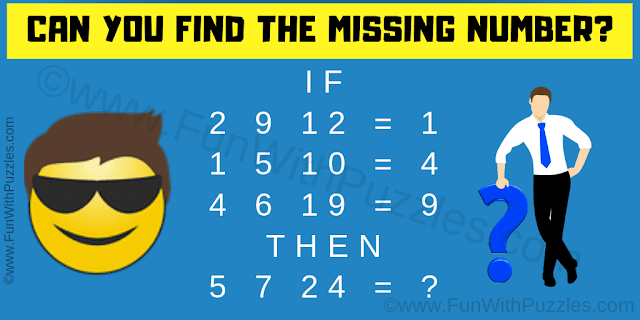This logic math question will twist your brain. It will test your logical reasoning skills. In this math logic puzzle question, you need to find the logic used in these given logical equations. Once you are able to find the hidden logic used in these number equation, solve the last equation. So can you find the value of the missing number which will replace the question mark in the last equation?Can you solve this logic math question?
Answer of this "Logic Math Question", can be viewed by clicking on the answer button.